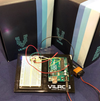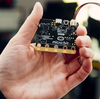0

## Defuse the Bomb Arduino Style### Diffuse the Bomb Project

In this project, we will use the Arduino Uno to control an LCD screen and 4x4 keypad. The keypad will be used to enter a password, time limit, then check user input against the set password. This will serve as a “Bomb” for games such as paintball, airsoft, etc.

### Hookup Instructions

1. Connect a jumper wire from the 5V pin on the Arduino to one of the + rails on the breadboard.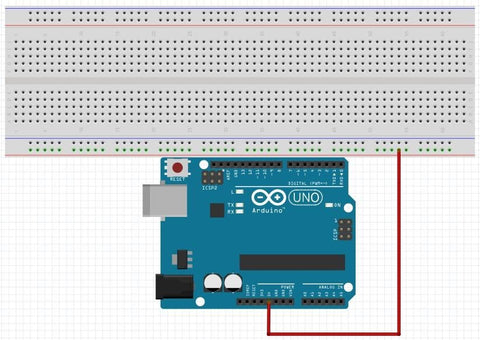2. Connect a jumper wire from the GND pin on the Arduino to the - rail next to the + rail you chose on the breadboard.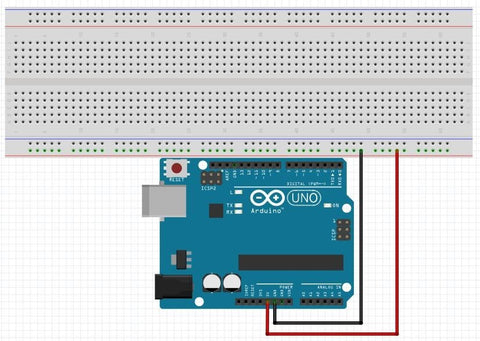3. Connect the keypad with the pins running along the lettered rows.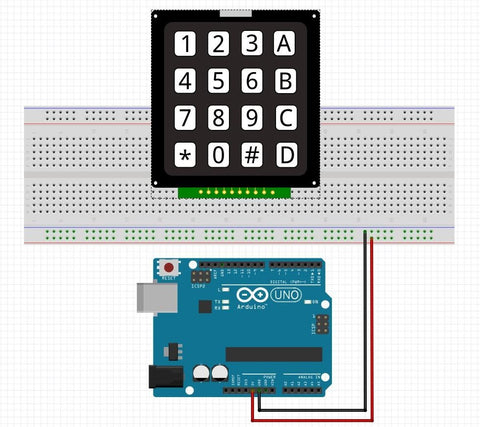4. Place jumper wires from pins 7-13 to the pins of the keypad.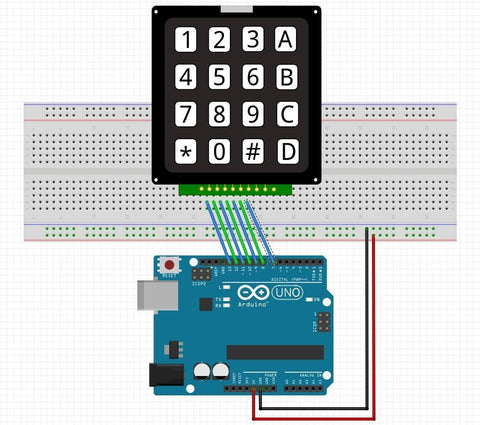5. Place 10 kohm resistors from the - rail with the GND pin connected to it to the first 4 pins from the left of the Keyboard.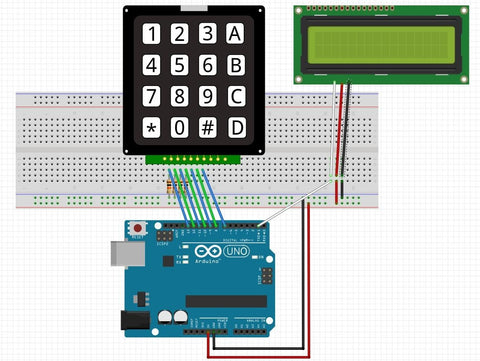### Programming Instructions

1. Overview: This project will ask for a 4 digit password and a time limit upon powering on the Arduino. The user will enter these via the 4x4 keypad. The keypad works by applying power to the column pins one at a time then checking if any of the row pins input goes high. If a button is pressed on a row, the power from the column is completed to the row pin, showing a high input on that pin and allowing you to see which button was pressed. You then assign a character value to that input combination. For example, if column 1 output is high and row 2 input goes high, then the ‘4’ button was pressed.
2. To begin with, we’ll need to declare all our variables that we’ll need.

// Initialize variables

int row_1 = 13;                                               // Row 1 is Pin 13

int row_2 = 12;                                               // Row 2 is Pin 12

int row_3 = 11;                                                // Row 3 is Pin 11

int row_4 = 10;                                                // Row 4 is Pin 10

int col_1 = 9;                                                // Column 1 is Pin 9

int col_2 = 8;                                                // Column 2 is Pin 8

int col_3 = 7;                                                // Column 3 is Pin 7

int col_4 = 6;                                                // Column 4 is Pin 6

int row = -1;                  // Variable to hold which row the button

pressed is on

int col = 0;                // Variable to hold which column the button

pressed is on

// This 4x4 matrix will represent each key on the keypad

char keypad = { {'1', '2', '3', 'A'},

{'4', '5', '6', 'B'},

{'7', '8', '9', 'C'},

{'*', '0', '#', 'D'}

};

char current_key;     // Variable to hold the current key pressed

char live_code;        // Variable to hold the user's live password

tries

int code_char = 0;       // Variable that holds what number of the password you're on

char time_arr = {0, 0, 0, 0}; // Variable to hold individual digits of time limit

char time_arr2 = {0, 0, 0, 0}; // Variable to hold reverse order of numbers input

int count;                                     // Number of digits in time limit

int time_limit = 0;   // Variable to store time limit (milli Seconds)

int etime = 0;                       // Variable to store elapsed time

int cur_time = 0;     // Variable to store current time

bool done = false;       // Variable to turn on when setup is done

3. Next, we’ll need to setup the LCD screen, tell the Arduino which pin is an input and which pin is an output, and ask the user for the password and time limit.  These steps only need to be done once, so we’ll put them into the setup routine.

void setup() {

cur_time = millis();                                 // Store the current time

// Set up our LCD screen

Serial.begin(9600);                     // Initialize Serial at 9600 baud

Serial.write(17);                          // Turn on the back light

Serial.write(24);        // Turn the display on, with cursor and no blink

Serial.write(12);                               // Clear the screen

Serial.write(128);                   // Move cursor to top left corner

// Set the pin modes

pinMode(row_1, INPUT);            // Make row 1 pin an input pinMode(row_2, INPUT);            // Make row 2 pin an input pinMode(row_3, INPUT);            // Make row 3 pin an input pinMode(row_4, INPUT);            // Make row 4 pin an input

pinMode(col_1, OUTPUT); // Make col 1 pin an output pinMode(col_2, OUTPUT); // Make col 2 pin an output pinMode(col_3, OUTPUT); // Make col 3 pin an output pinMode(col_4, OUTPUT); // Make col 4 pin an output

// Tell the user to enter the password

Serial.print("Enter 4 digit");

Serial.write(148);     // Move to second row

Serial.write(168);     // Move to third row

// This for loop loops through 5 times to get the four digit password and # to submit it

for (int i = 0; i < 5; i++) {

Serial.print(current_key);// Print it out

button_bounce();// Keep from reading the buttons more than once

// If you're on the last iteration, make sure you get the # symbol and nothing else

// Otherwise, reset taking the password

if (i == 4 && password[i] != '#') {

i = 0;                             // Set i back to 0

Serial.write(168);         // Move to the beginning of the third row

Serial.print("  ");           // Clear the third row

Serial.write(168);         // Move back to the beginning of the row

}

}

Serial.write(188);     // Move to the fourth row

for (int i = 0; i < 4; i++) { // Loop through the four digits of password

}

delay(2000);

// Tell the user to enter the time in minutes followed by # Serial.write(12);

Serial.write(128);

Serial.print("Enter time (min)");

Serial.write(148);

Serial.print("Followed by #");

Serial.write(168);

count = 0;                                     // Set count to 0

// Loop through 5 times to read up to a 4 digit time and #

for (int i = 0; i < 5; i++) {

// Make sure the input is a number that can be used in a time

if (current_key != 'A' && current_key != 'B' && current_key != 'C' && current_key != 'D' &&

current_key != '*' && current_key != '#') {

time_arr[i] = current_key; // If it is, store it in time_arr

count++;

// Increment count to keep track of how many digits are in the time

Serial.print(current_key); // Print the current input

button_bounce();                     // Keep from reading the button more than once

}

// If the key pressed isn't a number, tell the user

else if (current_key == 'A' || current_key == 'B' || current_key == 'C' || current_key == 'D' ||

current_key == '*') {

Serial.print("That's not a number!");

Serial.write(168);

delay(2000);

Serial.print("                                         ");

Serial.write(168);

}

// If the key pressed is # and it's not the first button, then put i to 5 to end the loop

else if (current_key == '#' && count != 0) {

Serial.print(current_key);   // Print the current key

button_bounce();                          // Keep from reading the button more than once

i = 5;

}

else {}                                           // Otherwise, do nothing

// If you're on the last iteration and the user doesn't input #, start the loop over

if (i == 4 && time_arr[i] != '#') {

i = 0;

}

}

time_limit = 0;                               // Set time_limit to 0, it's getting assigned 35 somewhere...

// Convert the chars to ints

for (int i = 0; i < count; i++) {

time_arr[i] = time_arr[i] - 48; // Subtracting 48 converts the ASCII value of numbers to the decimal value

// of those numbers (48 ASCII is '0', 49 is '1', etc)

}

// This takes each digit of the input time and multiplies it by what decimal place it holds

// I.E. If the user enters 12, then the 1 needs to be multiplied by 10 and the 2 by 1 then those two

// results added together to get 12. Count is how many digits there were, and 10^(count -1) is the value

// of the decimal place of each digit. Max(count - x, 0) takes whichever number is higher between

// count - x or 0 to make sure we don't use a negative power when, say, only 2 numbers were input.

time_limit = time_arr * pow(10, count - 1) + time_arr * pow(10, max(count - 2, 0)) +

time_arr * pow(10, max(count - 3, 0)) + time_arr * pow(10, max(count - 4, 0));

// Print the time limit in minutes

Serial.write(188);

Serial.print("Time Limit:");

Serial.print(time_limit);

Serial.print("min");

time_limit = time_limit * 60;         // Convert minute to seconds

delay(2000);

cur_time = millis();                      // Store the current time

Serial.write(12);                         // Clear the screen

Serial.write(128);                      // Go to top left corner

Serial.print("Time Left: ");         // Print the time left in seconds

Serial.print(time_limit);

Serial.print("sec");

delay(2000);

done = true;                                // Turn done on so the readpad routine will break every second to keep the timer working

code_char = 0;                            // Set code_char to 0

}

4. In the loop routine, we’ll read any input from the keypad and compare it with the password that was received during setup. If a wrong digit is input, start over taking a new password try. We’ll also need to keep track of how much time has passed and update the time remaining to output to the LCD.

oid loop() {

button_bounce();                       // Keep the button from being read more than once

if (live_code == 0) {}                  // If live_code is null, do nothing

// If live_code isn't the same as the index of password you're on, tell the user and start over taking

else if (live_code != password[code_char] && live_code != 0) {

Serial.write(148);

Serial.print("Incorrect digit!");

delay(1000);

Serial.write(148);

Serial.print("                              ");

code_char = 0;

}

// If live_code is a correct digit of password, go to taking the next digit

else if (live_code == password[code_char]) {

Serial.write(148 + code_char);

Serial.print(live_code);

code_char++;

}

// Otherwise, do nothing

else {}

// If you're on the last digit and it's correct, tell the user they won.

if (code_char == 4) {

Serial.write(148);

Serial.print("Bomb Diffused!");

Serial.write(168);

Serial.print("You win!!");

while (1) {}                                    // End the program

}

}

5. Here, you’ll notice a couple of routines I created - readpad() and button_bounce(). Creating a subroutine like this keeps you from having to type out long lists of commands each time you want to perform a repetitive task. Readpad() reads the input from the keypad, and button_bounce() freezes the program after the first time a button is pressed until it is released again. This is because the Arduino cycles in thousandths of a second, so even if you press the button really fast, it could see it several times!

int i = 0;

while (row == -1) {            // While row is -1, read the keypad

etime = millis() - cur_time;                // Calculate elapsed time (for after password and time have been stored)

if (etime >= 1000 && done == true) {

live_code = 0;                                 // Value to check against to show no key pressed

return live_code;

}

// Move between which column is high

if (i == 0) {

col = 0;                                                          // Set column to 1

digitalWrite(col_1, HIGH);                            // Turn column 1 on

digitalWrite(col_2, LOW);                            // Turn column 2 off

digitalWrite(col_3, LOW);                           // Turn column 3 off

digitalWrite(col_4, LOW);                            // Turn column 4 off

}

else if (i == 1) {

col = 1;                      // Set column to 2

digitalWrite(col_1, LOW);             // Turn column 1 off digitalWrite(col_2, HIGH);            // Turn column 2 on digitalWrite(col_3, LOW);             // Turn column 3 off digitalWrite(col_4, LOW);             // Turn column 4 off

}

else if (i == 2) {

col = 2;                      // Set column to 3

digitalWrite(col_1, LOW);            // Turn column 1 off digitalWrite(col_2, LOW);            // Turn column 2 off digitalWrite(col_3, HIGH);            // Turn column 3 on digitalWrite(col_4, LOW);            // Turn column 4 off

}

else if (i == 3) {

col = 3;                      // Set column to 4

digitalWrite(col_1, LOW);            // Turn column 1 off digitalWrite(col_2, LOW);            // Turn column 2 off digitalWrite(col_3, LOW);            // Turn column 3 off digitalWrite(col_4, HIGH);           // Turn column 4 on

}

else {

col = -1;

}

if ( i >= 3) {

i = 0;

}

else {

i++;

}

// Read the row of the button pressed                                        if (digitalRead(row_1) == HIGH) {

row = 0;    // If the row 1 pin is pressed, set row = 1

}

else if (digitalRead(row_2) == HIGH) {

row = 1;    // If the row 2 pin is pressed, set row = 2

}

else if (digitalRead(row_3) == HIGH) {

row = 2;    // If the row 3 pin is pressed, set row = 3

}

else if (digitalRead(row_4) == HIGH) {

row = 3;    // If the row 4 pin is pressed, set row = 4

}

else {

row = -1;    // If no pin is high, set row = 0

}

}

// Set the current key to the location in the matrix

// corresponding to the row and column read above current_key = keypad[row][col];

row = -1;

return current_key;                                 // Return the current

key as you exit the routine

}

// This keeps from reading a button more than once.     If a row input is high, freeze here until it goes

// low, then give it 250 milliseconds to make sure the user doesn't bounce the button

void button_bounce() {

delay(250);

}

}

6. You’re all done!

Compile and upload then have fun!

You can LEDs to light up if time expires if that’s desired! Just put the code to turn them on after the line that prints “You Lose!” and before while(1){}.

### Also in Raspberry Pi and Arduino Projects